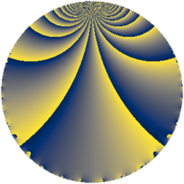# Properties

 Label 9800.2.a.crLevel $9800$ Weight $2$ Character orbit 9800.a Self dual yes Analytic conductor $78.253$ Analytic rank $0$ Dimension $4$ CM no Inner twists $2$

# Related objects

## Newspace parameters

 Level: $$N$$ $$=$$ $$9800 = 2^{3} \cdot 5^{2} \cdot 7^{2}$$ Weight: $$k$$ $$=$$ $$2$$ Character orbit: $$[\chi]$$ $$=$$ 9800.a (trivial)

## Newform invariants

 Self dual: yes Analytic conductor: $$78.2533939809$$ Analytic rank: $$0$$ Dimension: $$4$$ Coefficient field: $$\Q(\sqrt{7}, \sqrt{15})$$ Defining polynomial: $$x^{4} - 11 x^{2} + 4$$ Coefficient ring: $$\Z[a_1, a_2, a_3]$$ Coefficient ring index: $$2$$ Twist minimal: yes Fricke sign: $$-1$$ Sato-Tate group: $\mathrm{SU}(2)$

## $q$-expansion

Coefficients of the $$q$$-expansion are expressed in terms of a basis $$1,\beta_1,\beta_2,\beta_3$$ for the coefficient ring described below. We also show the integral $$q$$-expansion of the trace form.

 $$f(q)$$ $$=$$ $$q + \beta_{2} q^{3} + ( 2 - \beta_{3} ) q^{9} +O(q^{10})$$ $$q + \beta_{2} q^{3} + ( 2 - \beta_{3} ) q^{9} + q^{11} + ( \beta_{1} + \beta_{2} ) q^{13} + \beta_{1} q^{17} + ( -\beta_{1} + 2 \beta_{2} ) q^{19} + ( 2 - \beta_{3} ) q^{23} + ( \beta_{1} + 4 \beta_{2} ) q^{27} + \beta_{3} q^{29} + ( -\beta_{1} + \beta_{2} ) q^{31} + \beta_{2} q^{33} + ( -2 + \beta_{3} ) q^{37} + ( 6 - 2 \beta_{3} ) q^{39} -3 \beta_{2} q^{41} + ( -6 + \beta_{3} ) q^{43} + 2 \beta_{2} q^{47} + ( 1 - \beta_{3} ) q^{51} -2 \beta_{3} q^{53} + ( 9 - \beta_{3} ) q^{57} + ( \beta_{1} + \beta_{2} ) q^{59} + ( \beta_{1} - 3 \beta_{2} ) q^{61} + ( 3 + 2 \beta_{3} ) q^{67} + ( \beta_{1} + 7 \beta_{2} ) q^{69} + ( 8 + \beta_{3} ) q^{71} -5 \beta_{2} q^{73} -\beta_{3} q^{79} + ( 15 - 2 \beta_{3} ) q^{81} + ( -2 \beta_{1} + \beta_{2} ) q^{83} + ( -\beta_{1} - 5 \beta_{2} ) q^{87} + ( 2 \beta_{1} - \beta_{2} ) q^{89} + 4 q^{93} + ( -\beta_{1} + 5 \beta_{2} ) q^{97} + ( 2 - \beta_{3} ) q^{99} +O(q^{100})$$ $$\operatorname{Tr}(f)(q)$$ $$=$$ $$4q + 10q^{9} + O(q^{10})$$ $$4q + 10q^{9} + 4q^{11} + 10q^{23} - 2q^{29} - 10q^{37} + 28q^{39} - 26q^{43} + 6q^{51} + 4q^{53} + 38q^{57} + 8q^{67} + 30q^{71} + 2q^{79} + 64q^{81} + 16q^{93} + 10q^{99} + O(q^{100})$$

Basis of coefficient ring in terms of a root $$\nu$$ of $$x^{4} - 11 x^{2} + 4$$:

 $$\beta_{0}$$ $$=$$ $$1$$ $$\beta_{1}$$ $$=$$ $$($$$$\nu^{3} - 7 \nu$$$$)/2$$ $$\beta_{2}$$ $$=$$ $$($$$$\nu^{3} - 11 \nu$$$$)/2$$ $$\beta_{3}$$ $$=$$ $$\nu^{2} - 6$$
 $$1$$ $$=$$ $$\beta_0$$ $$\nu$$ $$=$$ $$($$$$-\beta_{2} + \beta_{1}$$$$)/2$$ $$\nu^{2}$$ $$=$$ $$\beta_{3} + 6$$ $$\nu^{3}$$ $$=$$ $$($$$$-7 \beta_{2} + 11 \beta_{1}$$$$)/2$$

## Embeddings

For each embedding $$\iota_m$$ of the coefficient field, the values $$\iota_m(a_n)$$ are shown below.

For more information on an embedded modular form you can click on its label.

Label $$\iota_m(\nu)$$ $$a_{2}$$ $$a_{3}$$ $$a_{4}$$ $$a_{5}$$ $$a_{6}$$ $$a_{7}$$ $$a_{8}$$ $$a_{9}$$ $$a_{10}$$
1.1
 0.613616 3.25937 −3.25937 −0.613616
0 −3.25937 0 0 0 0 0 7.62348 0
1.2 0 −0.613616 0 0 0 0 0 −2.62348 0
1.3 0 0.613616 0 0 0 0 0 −2.62348 0
1.4 0 3.25937 0 0 0 0 0 7.62348 0
 $$n$$: e.g. 2-40 or 990-1000 Significant digits: Format: Complex embeddings Normalized embeddings Satake parameters Satake angles

## Atkin-Lehner signs

$$p$$ Sign
$$2$$ $$1$$
$$5$$ $$1$$
$$7$$ $$-1$$

## Inner twists

Char Parity Ord Mult Type
1.a even 1 1 trivial
7.b odd 2 1 inner

## Twists

By twisting character orbit
Char Parity Ord Mult Type Twist Min Dim
1.a even 1 1 trivial 9800.2.a.cr yes 4
5.b even 2 1 9800.2.a.cq 4
7.b odd 2 1 inner 9800.2.a.cr yes 4
35.c odd 2 1 9800.2.a.cq 4

By twisted newform orbit
Twist Min Dim Char Parity Ord Mult Type
9800.2.a.cq 4 5.b even 2 1
9800.2.a.cq 4 35.c odd 2 1
9800.2.a.cr yes 4 1.a even 1 1 trivial
9800.2.a.cr yes 4 7.b odd 2 1 inner

## Hecke kernels

This newform subspace can be constructed as the intersection of the kernels of the following linear operators acting on $$S_{2}^{\mathrm{new}}(\Gamma_0(9800))$$:

 $$T_{3}^{4} - 11 T_{3}^{2} + 4$$ $$T_{11} - 1$$ $$T_{13}^{2} - 28$$ $$T_{19}^{4} - 71 T_{19}^{2} + 1024$$ $$T_{23}^{2} - 5 T_{23} - 20$$

## Hecke characteristic polynomials

$p$ $F_p(T)$
$2$ $$T^{4}$$
$3$ $$4 - 11 T^{2} + T^{4}$$
$5$ $$T^{4}$$
$7$ $$T^{4}$$
$11$ $$( -1 + T )^{4}$$
$13$ $$( -28 + T^{2} )^{2}$$
$17$ $$144 - 39 T^{2} + T^{4}$$
$19$ $$1024 - 71 T^{2} + T^{4}$$
$23$ $$( -20 - 5 T + T^{2} )^{2}$$
$29$ $$( -26 + T + T^{2} )^{2}$$
$31$ $$64 - 44 T^{2} + T^{4}$$
$37$ $$( -20 + 5 T + T^{2} )^{2}$$
$41$ $$324 - 99 T^{2} + T^{4}$$
$43$ $$( 16 + 13 T + T^{2} )^{2}$$
$47$ $$64 - 44 T^{2} + T^{4}$$
$53$ $$( -104 - 2 T + T^{2} )^{2}$$
$59$ $$( -28 + T^{2} )^{2}$$
$61$ $$( -60 + T^{2} )^{2}$$
$67$ $$( -101 - 4 T + T^{2} )^{2}$$
$71$ $$( 30 - 15 T + T^{2} )^{2}$$
$73$ $$2500 - 275 T^{2} + T^{4}$$
$79$ $$( -26 - T + T^{2} )^{2}$$
$83$ $$100 - 155 T^{2} + T^{4}$$
$89$ $$100 - 155 T^{2} + T^{4}$$
$97$ $$16384 - 284 T^{2} + T^{4}$$